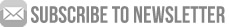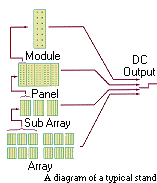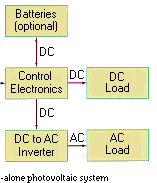ABOUT US INFORMATION PANELS PRODUCTS PROJECTS DESIGN SHOP

Solar System Design Shop

Design and Installation of PV System

The selection and proper installation of appropriately-sized components directly affect system reliability, lifetime, and initial cost. In any installation, it must be kept in mind that trade-offs are necessary in system design and component selection. Information provided here will assist in making your own system decisions.

Systems may be divided into three types, and design and installation of each type encompasses unique considerations and concerns:
• Stand-alone systems - those systems which use photovoltaics technology only, and are not connected to a utility grid.

• Hybrid systems - those systems which use photovoltaics and some other form of energy, such as diesel generation or wind.

• Grid-tied systems - those systems which are connected to a utility grid.You must estimate the amount of power required by the load. This is done by listing each load and making an estimate for how energy it will consume in a day. The worksheet calculations will yield the total daily load given in Amp-hours. If the load demand varies widely from month-to-month (or season-to-season), you must fill out Worksheet #1 for each month. Usually the system size will be dictated by the worst-case month; the month with the largest load and the lowest solar insolation. Consider this month first.
Each box in the worksheet is numbered. The instructions below tell you how to fill in each box.

1 Load Description: List each load (i.e., fluorescent lamp, pump, radio). Enter the dc loads at the top and the ac loads, if any, at the bottom part of the worksheet.
2 QTY: Enter the number of identical loads in the system.
3 Load Current (A): Enter an estimate of the current required by each load when operating. Use the manufacturer's rated current, or measure the current.
4 Load Voltage (V): Enter the voltage of the load, i.e., 120 volt ac, 24 volt dc, etc. The operating voltage is usually listed on the appliance.
5A DC Load Power (W): Calculate and enter the power required by the dc load. Power equals the product of voltage and current.
5B AC Load Power (W): Calculate and enter the power required by the ac load. Ignore the power factor for this calculation.
6 Daily Duty Cycle (hrs/day): Enter the average amount of time per day the load will be used. (Enter fractions of hours in decimal form, i.e., 1 hour, 15 minutes would be entered as 1.25.)
7 Weekly Duty Cycle (days/wk): Enter the average number of days each week the load will be used.
8 Power Conversion Efficiency (decimal): This factor accounts for power loss in systems using power conditioning components (converters or inverters). If the appliance requires ac power or dc power at a voltage other than your system voltage, you should enter the conversion efficiency of the device. If you do not have the actual efficiency of the converter being used, use the default values given below for initial sizing.

 Power Conversion Efficiency Default DC to AC 0.85 DC to DC 0.9

9 Nominal System Voltage (V): Enter the desired system voltage. The system voltage is normally the voltage required by the largest loads. Common values are 12 or 24 volts dc and 120 volts ac.
10 Amp-Hour Load (Ah/day): Calculate the average energy requirement per day in ampere-hours by performing the calculations as indicated by the mathematical symbols across the page.
11 Total DC and AC Load Power (W): Enter the individual ac and/or dc loads.
11A Total dc load in Watts.
11B Total ac load in Watts.
12 Total Ampere-Hour Load (Ah/day): Calculate the daily average system load in ampere-hours.
13 Total DC Load Power (W): Enter value from Block 11A.
14 Total AC Load Power (W): Enter value from Block 11B.
15 Nominal System Voltage (V): Enter value from Block 9.
16 Peak Current Draw (A): Calculate the maximum current required if all the loads are operating simultaneously. This value is used for sizing fuses, wiring, etc.
17 Total Ampere-Hour Load (Ah/day): Enter value from Block 12.
18 Wire Efficiency Factor (decimal) (1 - wire loss): Enter the decimal fraction accounting for loss due to wiring and switchgear. This factor can vary from 0.95 to 0.99. Wire size should be chosen to keep wire loss in any single circuit to less than 3 percent (>0.97).

Wire Efficiency Factor Default Value = 0.98

19 Battery Efficiency Factor (decimal): Enter the battery efficiency which is equal to ampere-hours out divided by ampere-hours in. Use manufacturer's data for specific battery. Assume constant voltage operation.

 Battery Efficiency Factor Default Value = 0.90

20 Corrected Amp-Hour Load (Ah/day): Calculate the energy required to meet the average daily load plus losses.
(2) Design Current and Tilt Angle

(Picture: Current design.gif)
Design Current and the optimum tilt angle for your PV array. An array set at the same angle as the latitude of the site will receive the maximum annual solar radiation. If the load demand is high in the winter (northern hemisphere), set the array tilt at latitude plus 15°. For a predominant summer load, set the array tilt angle at latitude minus 15°. Calculate the design current for all three tilt angles if the load demand varies widely throughout the year. Completing the calculation on the worksheet will yield the optimum tilt angle for your array. The numbered instructions correspond to the numbers in the boxes in the worksheet.

21 System Location/Insolation Location: Enter the latitude and longitude of the system site and the location of the insolation data used. See Appendix A.
22A, B, & C Corrected Load (Ah/day): See Block 20 Worksheet #1. Enter the corrected load for each month for each tilt angle.
23A, B, & C Peak Sun (hrs/day): Enter the average number of hours each day when insolation was 1,000 watts per square meter. Enter the value for each month for each tilt angle. Weather data for selected sites is given in Appendix A.

 NOTE: Peak sun hours are equal to the average kilowatt-hours/m2-day. 1 kwh/m2 = Langley/85.93 = 316.96 Btu/ft2 = 3.6 MJ/m2.

24A, B, & C Design Current (A):
Calculate the current required to meet the system load.

 NOTE: The recommended tilt angle for the array is selected by first determining the largest design current for each of the three tilt angles; then selecting the smallest of those three values.

25A + 26A Peak Sun (hrs/day) and Design Current (A): Select and enter the largest monthly design current and corresponding peak sun hours from columns 24A, 24B, and 24C.
27 & 28 Peak Sun (hrs/day) and Design Current (A): Select and enter the smallest of the three design currents and the corresponding peak sun hours from 25A, B, or C and 26A, B, or C.
(3) Battery Size
(Picture: Design Battery .gif)
29 Corrected Amp-Hour Load (Ah/day): Enter value from Block 20 Worksheet #1.
30 Storage Days: Choose and enter the consecutive number of days the battery subsystem is required to meet the load with no energy production by the array. System availability is defined as critical (99 percent available) or non critical (95 percent available) and directly affects the number of storage days. Use the chart below to find the recommended number of storage days if no better estimate can be made.
31 Maximum Depth of Discharge (decimal): Enter the maximum discharge allowed for the battery. This depends on size and type of battery. Consult the battery manufacturer or use default values below.

 Maximum Depth of Discharge Battery Type Default Value Lead Acid Shallow Cycle 0.25 Lead Acid Deep Cycle 0.75 Nickel Cadmium 0.90

32 Derate for Temperature (decimal): Enter a factor that derates the battery capacity for cold operating temperatures. Ask the battery manufacturer for this information. If no better information is available derate a lead acid battery's capacity one percent for each degree Celsius below 20°C operating temperature.

 Temperature Correction Factor Default = 0.90

33 Required Battery Capacity (Ah): Calculate the battery capacity required to meet the daily load for the required number of days.

 NOTE: Select a battery for your system and record the specifications in the battery information block.

34 Capacity of Selected Battery (Ah): Enter the manufacturer's rating of battery storage capacity in ampere-hours. Batteries are normally rated at optimum test conditions; 20°C, and discharge rates of C/20 or lower.
35 Batteries in Parallel: Calculate the number of parallel connected batteries required to provide the storage capacity.
36 Nominal System Voltage (V): Enter the value from Block 9, Worksheet #1.
37 Nominal Battery Voltage (V): Enter the rated voltage of the selected battery, i.e., 2, 6, or 12 volts.
38 Batteries in Series: Calculate the number of series connected batteries required to provide the system voltage.
39 Batteries in Parallel: Enter the value from Block 35.
40 Total Batteries: Calculate the total number of batteries in the system.
41 Batteries in Parallel: Enter the value from Block 35.
42 Capacity of Selected Battery (Ah): Enter the value from Block 34.
43 System Battery Capacity (Ah): Calculate the battery system storage capacity.
44 Maximum Depth of Discharge (decimal): Enter the value from Block 31.
45 Usable Battery Capacity (Ah): This is the amount of ampere-hours that can safely be used from the installed batteries.
(4) System array Size

Use Worksheet #4 to calculate the size of the photovoltaic array. This should be large enough to provide energy to the load during the Design Month and to fully recharge the batteries during periods of sunny weather.

46 Design Current (A): Enter the value from Block 28, Worksheet #2.
47 Module Derate Factor (decimal): Enter a factor to adjust module current from standard operating conditions (SOC) of 1,000 w/m2 and 45°C temperature to field conditions, i.e., dust accumulations, mismatch loss between modules, degradation over time, etc.) Ask the module distributor or use the default values below.

 Module Derate Factor Default Value Module Type Default Value Single or Polycrystalline Silicon 0.90 Amorphous Silicon 0.70

48 Derated Design Current (A): Calculate the minimum array current necessary to supply the average daily load at the chosen site.

 NOTE: Select a PV module and record the specifications in the module information block. Be sure to determine the module voltage when it is operating at the highest temperatures expected for your site.

49 Rated Module Current (A): Enter the rated module operating current at 1,000 w/m2 and 45°C operating temperature as given by the manufacturer.
50 Modules in Parallel: Calculate the number of parallel connected modules required to provide the array current.
51 Nominal Battery Voltage (V): Enter the value from Block 37 Worksheet #3.
52 Batteries in Series: Enter the value from Block 38 Worksheet #3.
53 Voltage Required to Charge Batteries (V): Calculate the minimum voltage required to charge the batteries.
54 Voltage at Highest Module Temperature (V): Enter this value from the manufacturer's specifications.
55 Modules in Series: Calculate the number of series connected modules required to produce the system voltage. You must not round down. Round up or select another module with a higher operating voltage.
56 Modules in Parallel: Enter the value from Block 50.
57 Total Modules: Calculate the total number of modules in the array.
58 Modules in Parallel: Enter value from Block 50.
59 Rated Module Current (A): Enter the module current when operating at 1,000 w/m2 and 45°C temperature.
60 Rated Array Current (A): Calculate the rated array current when operating at 1,000 w/m2 and 45°C temperature.
61 Module Short Circuit Current (A): Enter module short circuit current when operating at 1,000 w/m2 and 45°C temperature.
62 Array Short Circuit Current (A): Calculate the array short circuit current when operating at 1000 w/m2 and 45°C temperature.
63 Modules in Series: Use the value from Block 55.
64 Rated Module Voltage (V): Enter module voltage when operating at 1,000 w/m2 and 45°C temperature.
65 Array Rate Voltage (V): Calculate array voltage when operating at 1,000 w/m2 and 45°C temperature.
66 Open Circuit Module Voltage (V): Enter module open circuit voltage when operating at 1,000 w/m2 and 45°C temperature.
67 Array Open Circuit Voltage (V): Calculate array open circuit voltage when operating at 1,000 w/m2 and 45°C temperature.

 NOTE: In some applications you may wish to know the highest voltages that might be produced by the array. This will occur when the array is operating at its lowest temperature. Use manufacturer's data to determine module voltage for the coldest temperatures expected.
Projects Completed by Dusol
Shop # 30, Beniyas Bldg, Beniyas Road, Deira, Dubai, United Arab Emirates